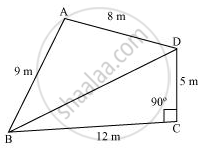# A park, in the shape of a quadrilateral ABCD, has ∠C = 90°, AB = 9 m, BC = 12 m, CD = 5 m and AD = 8 m. How much area does it occupy? - Mathematics

A park, in the shape of a quadrilateral ABCD, has ∠C = 90°, AB = 9 m, BC = 12 m, CD = 5 m and AD = 8 m. How much area does it occupy?

#### Solution

Let us join BD.

In ΔBCD, applying Pythagoras theorem,

BD2 = BC2 + CD2

= (12)2 + (5)2

= 144 + 25

BD2 = 169

BD = 13 mArea of ΔBCD

= 1/2xxBCxxCD = (1/2xx12xx5)m^2=30m^2

For ΔABD,

s="Perimeter"/2=(9+8+12)/2=15m

By Heron's formula,

"Area of triangle "=sqrt(s(s-a)(s-b)(s-c))

"Area of "triangleABD=[sqrt(15(15-9)(15-8)(15-13))]m^2

=(sqrt(15xx6xx7xx2))m^2

=6sqrt35 m^2

= (6 x 5.916) m2

= 35.496 m2

Area of the park = Area of ΔABD + Area of ΔBCD

= 35.496 + 30 m

= 65.496 m2

= 65.5 m2 (approximately)

Concept: Application of Heron’s Formula in Finding Areas of Quadrilaterals
Is there an error in this question or solution?
Chapter 12: Heron's Formula - Exercise 12.2 [Page 206]

#### APPEARS IN

NCERT Class 9 Maths
Chapter 12 Heron's Formula
Exercise 12.2 | Q 1 | Page 206

Share